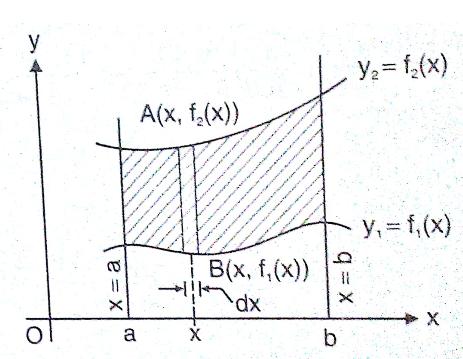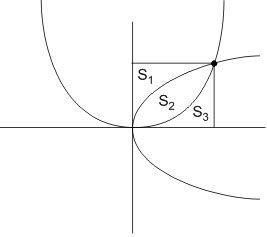# The parabolasdivide the square region bounded by the linesand the coordinate axes. Ifare respectively the areas of these parts numbered from top to bottom thenis Option 1)Option 2)Option 3)Option 4)As we learnt in

Area along x axis -

Letbe two curve then area bounded between the curves and the lines

x = a and x = b is- whereinWhereTotal area = S+ S+ S= 16

Also S= S3

ThusOption 1)This is incorrect option

Option 2)This is incorrect option

Option 3)This is correct option

Option 4)This is incorrect option